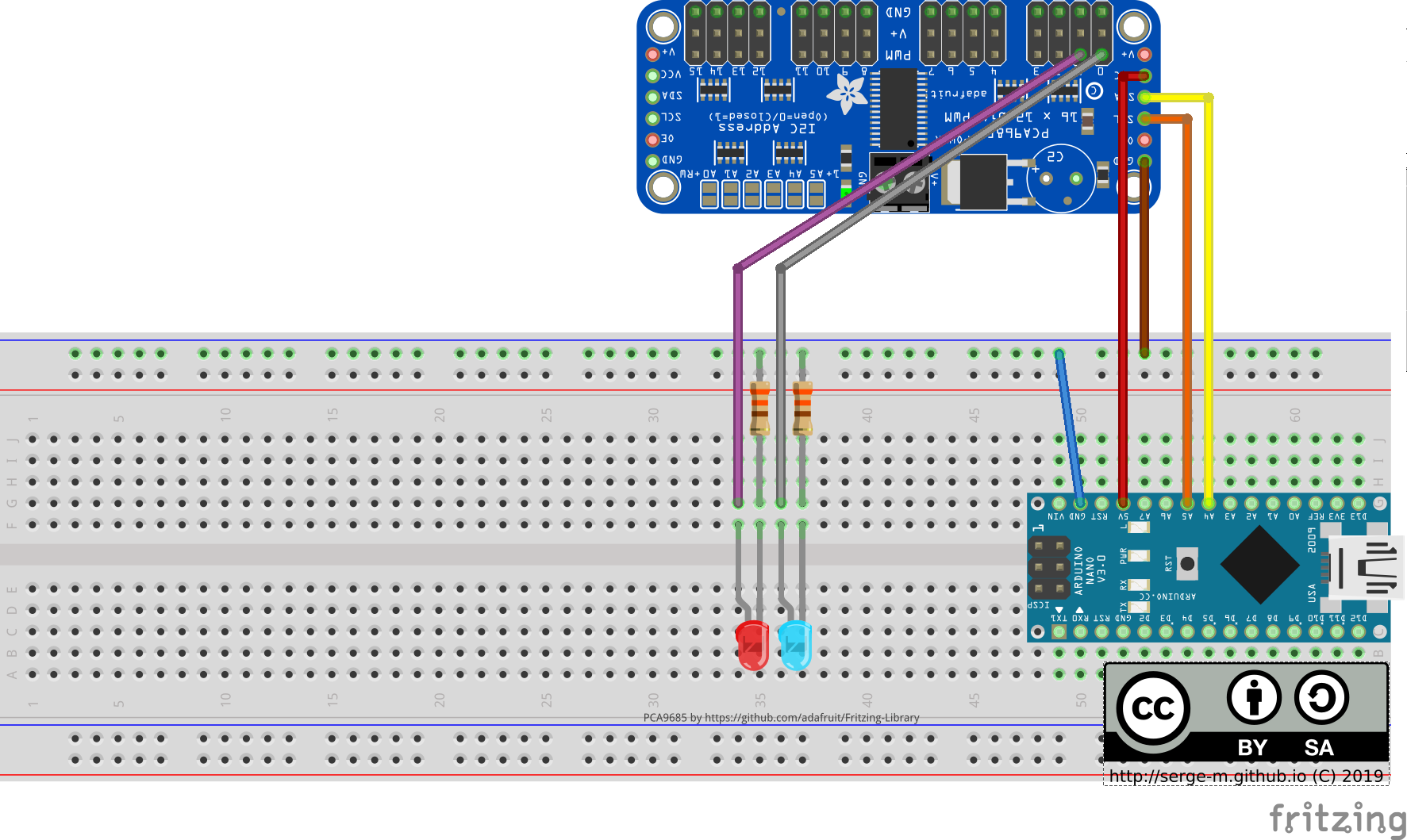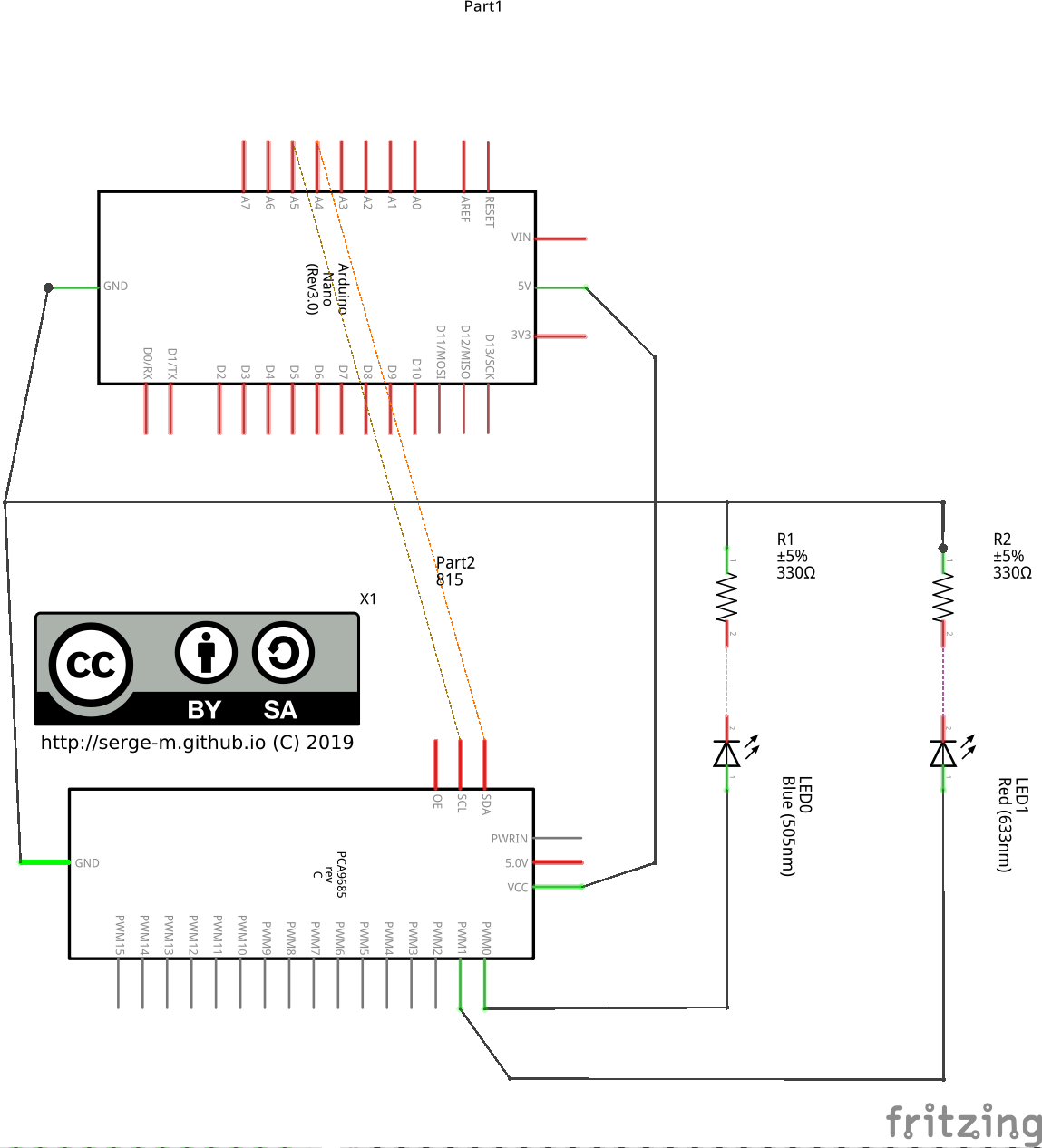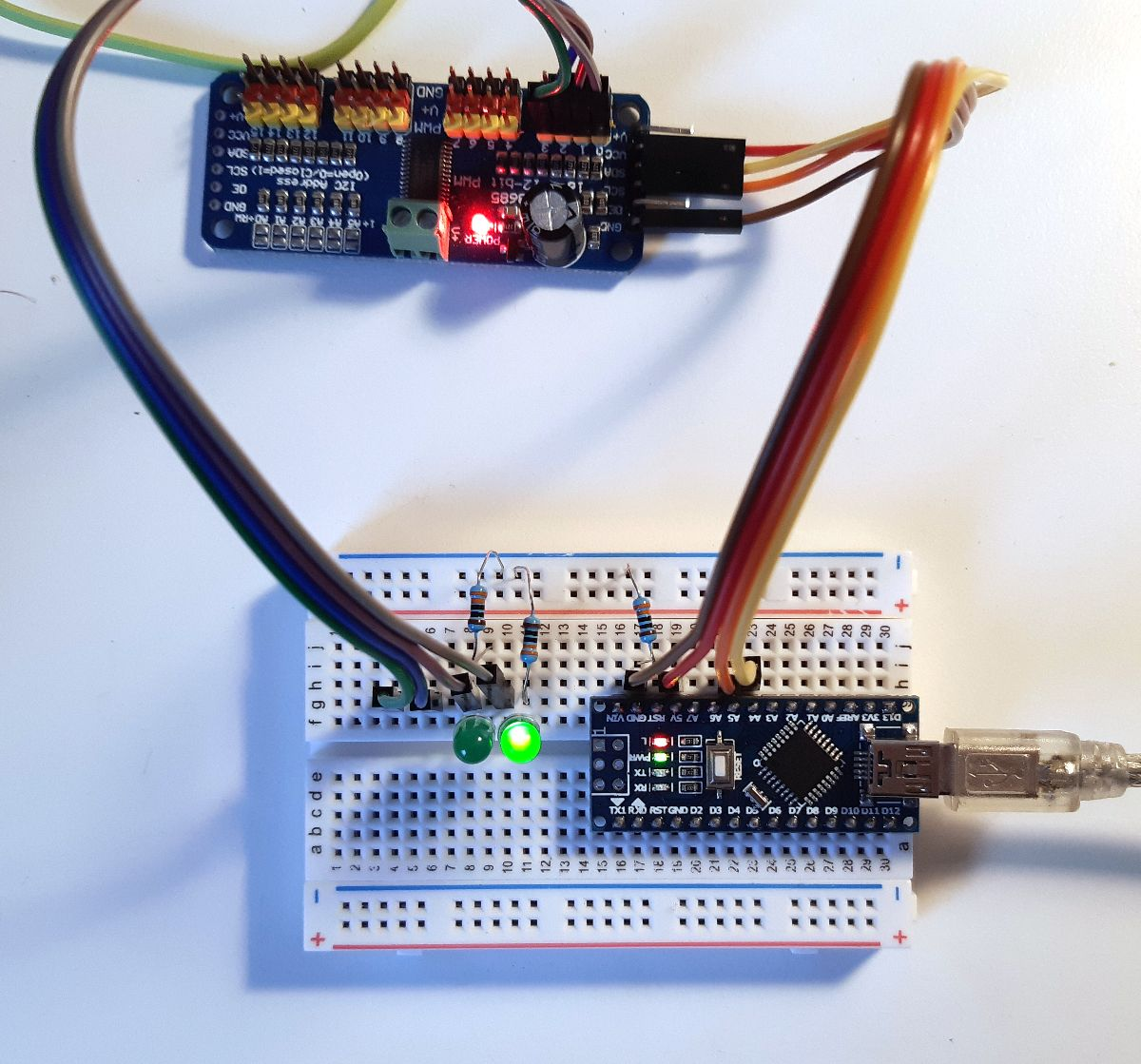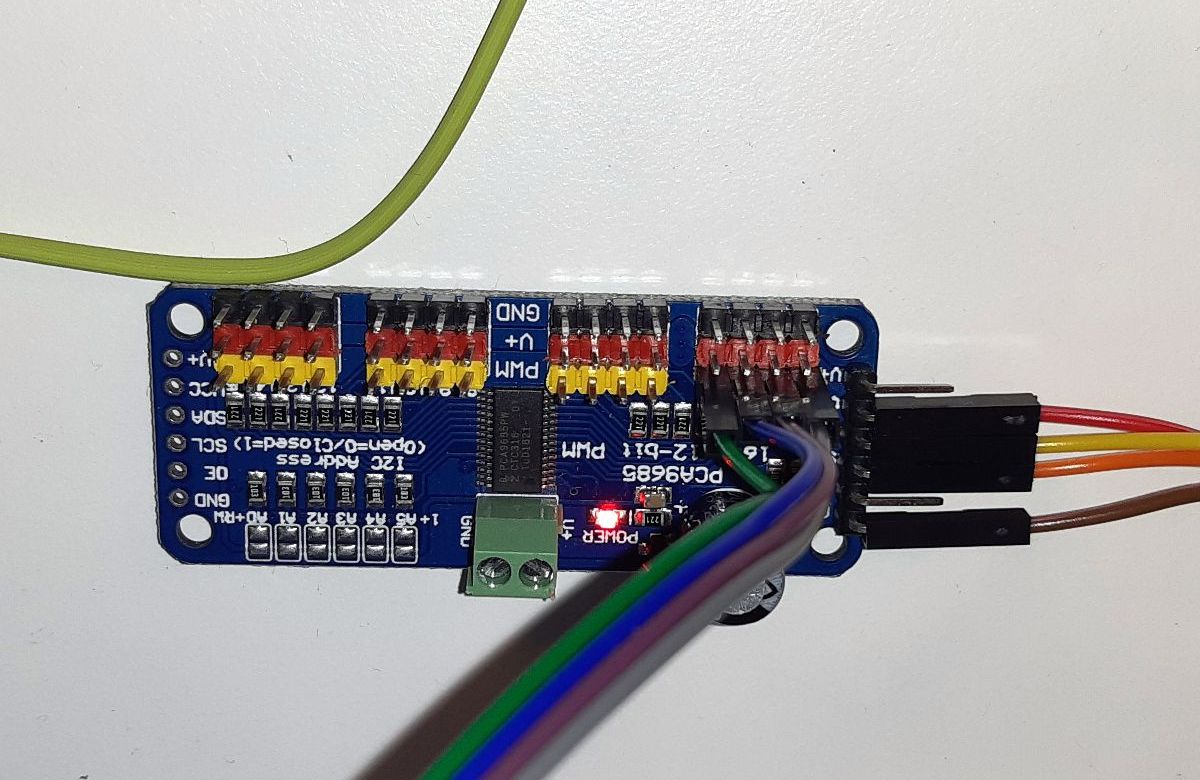Arduino (ATMega328p) boards can be used to control multiple LEDs. To simplify the management of the pulse width modulation and use only a couple of arduino's pins for many LEDs I used PCA9685 controller.

PCA9685 is connected to Arduino using I2C interface that requires only two data pins.Arduino connects to PCA9685 using `Adafruit_PWMServoDriver` library:

```#include <Adafruit_PWMServoDriver.h>

```

Initialization in `setup()`:

```pwm.begin();
pwm.setPWMFreq(1600);  // This is the maximum PWM frequency
```

Then for each loop we will blink with 2 LEDs. That can be easily extended to more LEDs:

Initialization in `setup()`:

```void loop() {
// set state 1
digitalWrite(LED_BUILTIN, HIGH);
pwm.setPWM(0, 0, 3072);
pwm.setPWM(1, 0, 4096);

// wait
delay(500);

// set state 2
digitalWrite(LED_BUILTIN, LOW);
pwm.setPWM(0, 0, 4096);
pwm.setPWM(1, 0, 4095);

// wait
delay(500);

}
```

`pwm.setPWM(p, start, end);` means that for the pin `p` the signal should transition from low to high at tick `start` (between 0..4095) and the signal should transition from high to low at tick `end` (between 0..4095).

There are special settings for full on and full off. Full on:

```pwm.setPWM(pin, 4096, 0);
```

Full off:

```pwm.setPWM(pin, 0, 4096);
```

Along with the other LED we will also control an LED that is built in the Arduino:

```void setup() {
pinMode(LED_BUILTIN, OUTPUT);
// ...
}

void loop() {
digitalWrite(LED_BUILTIN, HIGH); // LED on
// ...
digitalWrite(LED_BUILTIN, LOW);  // LED off
// ...
}
```

Here is the full listing:

```#include <Adafruit_PWMServoDriver.h>

void setup() {
pinMode(LED_BUILTIN, OUTPUT);

pwm.begin();
pwm.setPWMFreq(1600);  // This is the maximum PWM frequency
}

void loop() {
// set state 1
digitalWrite(LED_BUILTIN, HIGH);
pwm.setPWM(0, 0, 3072);
pwm.setPWM(1, 0, 4096);

// wait
delay(500);

// set state 2
digitalWrite(LED_BUILTIN, LOW);
pwm.setPWM(0, 0, 4096);
pwm.setPWM(1, 0, 4095);

// wait
delay(500);

}
```

Results:

One issue I had with that Adafruit_PWMServoDriver library is that it is blocking. If your PCA9685 is off or gets disconnected the whole program freezes. As far as I understand Adafruit_PWMServoDriver uses Wire as a backend and Wire is blocking. That is a known issues and there are some workarounds.